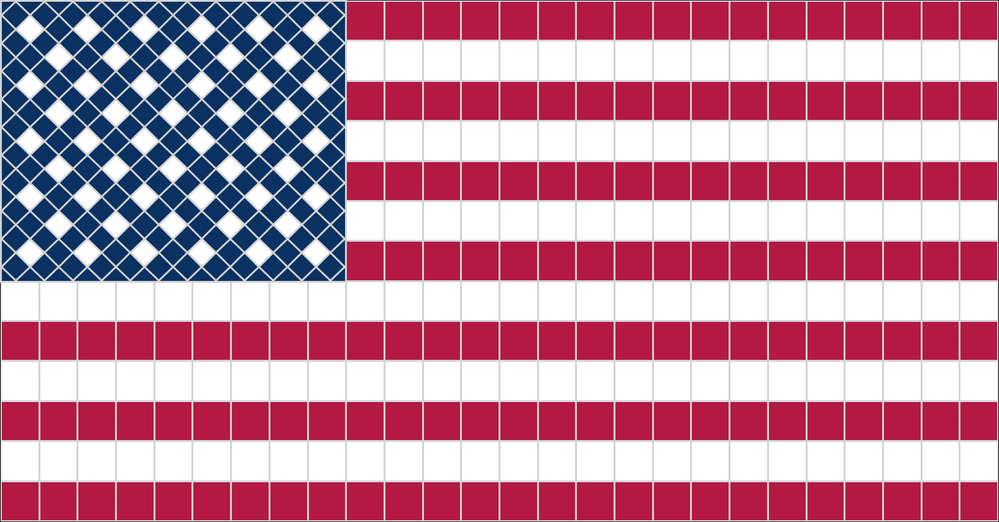## Fun With SAS ODS Graphics: Etsy-Inspired Mosaic Tile Squares USA FlagInspired by those clever mosaic flags on Etsy and Pinterest, here's a picture-in-picture SAS ODS Graphics imagining of what US flags might look like had Betsy Ross been a tile setter instead of a seamstress. Happy Fourth of July weekend, all!

``````* Fun With SAS ODS Graphics: Etsy-Inspired Mosaic Tile Squares USA Flag;

data stars(keep=id color x y rn cn);                                 /* Generate diamond-shped points for 50 stars (rotated squares, lengths fo sides=1 */
rn=-1;
do r=-.7071 to -.7071+12*1.414 by 1.414;                             /* Loop through rows (diamond height is sqrt(2)=1.414) */
rn+1; cn=-1;                                                       /* Looop through columns */
do c=-.7071 to -.7071+12*1.414 by 1.414;                           /* Odd rows have 6 stars, even have 5 (offset by 1) */
cn+1; if ((mod(rn,2) and mod(cn,2)) or (^mod(rn,2) and ^mod(cn,2))) then color=2; else color=1;
if cn=0 or cn>11 or rn<1 or rn>9 then color=1;                  /* Make 'padding' border 'Old Glory Blue' */
id+1; x=c-.7071; y=r; output; x+.7071; y+.7071; output;         /* Generate a polygon for diamonds - stars (white) and padding (blue) */
x+.7071; y+(-.7071); output; x+(-.7071); y+(-.7071); output;
end;
end;

ods listing gpath='/home/ted.conway/flag';                           /* Save generated image of stars and 'canton' (blue rectangle) */;
ods graphics / reset width=5.785in height=4.71in imagename='stars' antialias;
proc sgplot data=stars noautolegend nowall noborder pad=0 subpixel;  /* Generate 'canton' + stars */
styleattrs backcolor=cx0A3161 datacolors=(cx0A3161 white);           /* cx0A3161=Old Glory Blue */
polygon x=x y=y id=id / outline lineattrs=(thickness=2.5pt color=lightgrey) fill group=color; /* Diamond 'tiles' with light grey 'grout' */
xaxis display=none offsetmin=0 offsetmax=0 values=(-.7086 to 16.5624 by 1.414); /* Suppress and limit axes */
yaxis display=none offsetmin=0 offsetmax=0 values=(-.7071 to 13.4329 by 1.414);

data stripes(keep=id color x y rn);                                  /* Generate 13 red and white stripes */
rn=-1;
do r=-6*1.919-.7071 to -.7071+10*1.414 by 1.919;                     /* Note: Six stripes are same height as 'canton' */
rn+1;
if mod(rn,2) then color=2; else color=1;                           /* Alternate red/white stripes - red on top and bottom */
do c=-.7071 to 47.4699 by 1.919;                                   /* Generate red/white square polygons (length of sides=1.919) */
id+1; x=c; y=r; output; y+(1.919); output; x+1.919; output; y+(-1.919); output;
end;
end;

%SGANNO;                                                             /* Annotate larger stripes chart with smaller stars chart using SAS-provided macros */
data stars_anno;
%SGIMAGE (image="/home/ted.conway/flag/stars.png", border="TRUE", linecolor="lightgrey", linethickness=3,
anchor="topleft", width=17.26, widthunit="DATA", drawspace="datapercent", X1=0, Y1=100);
/* Save generated Stars and Stripes image */
ods graphics / reset width=16.742in height=8.7471in imagename='StarsAndStripes' antialias;
proc sgplot data=stripes noautolegend nowall noborder pad=0 sganno=stars_anno subpixel; /* Generate stripes chart annotated with stars chart (overlaid in upper left hand corner) */
styleattrs datacolors=(cxB31942 white);                             /* cxB31942='Old Glory Red' */
polygon x=x y=y id=id / outline lineattrs=(thickness=2.5pt color=lightgrey) fill group=color; /* 13 red and white stripes, light grey 'grout' */
xaxis display=none offsetmin=0 offsetmax=0 values=(-.7071 to 49.3889 by 1.919);    /* Suppress and limit axes */
yaxis display=none offsetmin=0 offsetmax=0 values=(-12.2211 to 13.4329 by 1.919);
run;``````